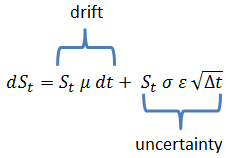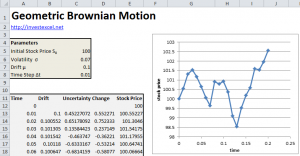# Simulate Geometric Brownian Motion with Excel

Stock prices are often modeled as the sum of

• the deterministic drift, or growth, rate
• and a random number with a mean of 0 and a variance that is proportional to dt

This is known as Geometric Brownian Motion, and is commonly model to define stock price paths.  It is defined by the following stochastic differential equation.

St is the stock price at time t, dt is the time step, μ is the drift, σ is the volatility,  Wt is a Weiner process, and ε is a normal distribution with a mean of zero and standard deviation of one .

Substituting Equation 2 into Equation 1 gives

Hence  dSt is the sum of a general trend, and a term that represents uncertainty.## Simulate Geometric Brownian Motion in Excel

Converting Equation 3 into finite difference form gives

Bear in mind that ε is a normal distribution with a mean of zero and standard deviation of one. This can be represented in Excel by NORM.INV(RAND(),0,1).

The spreadsheet linked to at the bottom of this post implements Geometric Brownian Motion in Excel using Equation 4.Simulate Geometric Brownian Motion in Excel### 5 thoughts on “Simulate Geometric Brownian Motion with Excel”

1. I need a free software for ornstein uhlembek, geometric Geometric Brownian Motion, jump diffusion, regime switching and mean reverting method

2. Have you tried the R library sde?

3. Hi Samir,
Wonderful website!
I’m trying to do a Brownian motion code for VBA.
But something doesn’t work.